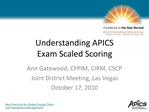DownloadDownload PresentationUnderstanding APICS Exam Scaled Scoring

# Understanding APICS Exam Scaled Scoring

Download Presentation## Understanding APICS Exam Scaled Scoring

- - - - - - - - - - - - - - - - - - - - - - - - - - - E N D - - - - - - - - - - - - - - - - - - - - - - - - - - -
##### Presentation Transcript

1. Understanding APICS Exam Scaled Scoring Ann Gatewood, CFPIM, CIRM, CSCP Joint District Meeting, Las Vegas October 17, 2010

2. Exam Items CPIM exams: 75 questions (BSCM is 105) CSCP exam: 175 questions 15/25 questions on every exam are Pre-test Not used for scoring Gathering statistics to ensure quality items Each item has a set of statistics to rate difficulty

3. 3 Exam Scores Exam scores are based on the number of questions answered correctly = raw score Raw score = score before any statistical techniques are used to refine or summarize. Each exam has a different difficulty, and a different passing raw score It is important that candidates answer all questions, since the score is based on the number of questions answered correctly.It is important that candidates answer all questions, since the score is based on the number of questions answered correctly.

4. 4 Passing Cut Score Study Determines the minimum raw score required to pass a particular exam Conducted when changes occur to module Panel of committee members, instructors, newly-certified individuals, international Each member analyzes each question to determine difficulty for candidate Two methods used To determine the minimum passing score, a cut score study is used. This involves the analysis and rating of each question for difficulty by a panel of experts.To determine the minimum passing score, a cut score study is used. This involves the analysis and rating of each question for difficulty by a panel of experts.

5. 5 Passing Cut Score Study Each question calibrated to scale at which the minimally qualified candidate has a probability of correctly answering the question. Estimates are averaged, and then summed to determine the passing cut score. Passing cut score is assigned to a point of difficulty instead of a number or % of correct responses. The panel rates each question on the scale at which the minimally qualified candidate has an even probability of correctly answering the question. The panel members scores are averaged. Once each question is rated by the panel, then we can sum these ratings to determine the cut score. The panel rates each question on the scale at which the minimally qualified candidate has an even probability of correctly answering the question. The panel members scores are averaged. Once each question is rated by the panel, then we can sum these ratings to determine the cut score.

6. Passing Cut Score Example - Angoff 69.05% of questions passed = raw passing score of 41.43 (60 question exam)

7. Passing Cut Score Example - Hofstee Using theoretical distribution, passing cut score = 67%, or 40.2 questions correct

8. Passing Cut Score Example

9. 9 Scaled Scoring Multiple versions of the exam are not equivalent due to variations in difficulty level. Technique for equating scores where the degree of difficulty varies from one exam to the next. The answer to this enigma is a technique called scaled scoring.The answer to this enigma is a technique called scaled scoring.

10. 10 Equating Adjusts the minimum raw passing score to compensate for these variations. Statistical process is used to convert the raw score to a scaled score. Equivalent passing standard for each exam is maintained. As we discussed earlier, multiple versions of the test do not have exactly the same difficulty due to variations in question difficulty. So the statistical technique of equating is used to offset these variations. Consequently, an equivalent passing standard for each exam is maintained.As we discussed earlier, multiple versions of the test do not have exactly the same difficulty due to variations in question difficulty. So the statistical technique of equating is used to offset these variations. Consequently, an equivalent passing standard for each exam is maintained.

11. Scaled Scoring Example

12. 12 APICS Exam Scaled Scoring Scale of CPIM tests ranges from 265 to 330 Scaled passing score is 300 Scale of CSCP tests ranges from 200 to 350 Scaled passing score is 300 The APICS scaled score ranges from 285 to 330, with a scaled passing point of 300.The APICS scaled score ranges from 285 to 330, with a scaled passing point of 300.